Next: Breit-Wheeler Process Up: Compton Process Previous: Usage

### Algorithm

The functions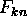(k=1,2) are stored in a 5-dimensional array FF(k,n,i,j,l) (n=1,MPH), (i=0,MY), (j=0,MXI), (l=0,MLM). The integral over y from 0 to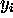is stored in FINT(k,n,i,j,l). The integral over the full range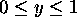is then FINT(k,n,MY,j,l) For integration, the trapezondal rule is used, which means the functionis approximated by a piecewise linear function. The sum of FINT(k,n,MY,j,l) over n is stored in FALL(k,j,l).

For a given initial condition, calculate the parameters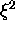and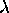and find FALL(*) by 2-dimensional interpolation. (The asterisk * indicates the appropriate sum over the initial polarization, i,e., FALL(*)=FALL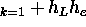FALL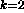). Then, calculate the total probability P (eq.(131) times the time interval DT):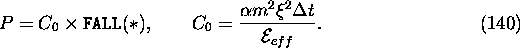Generate a uniform random number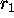in the interval (0,1). If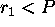, decide to emit a photon and, otherwise reject.

If rejected, the helicity of the electron should be changed, according to eq.(12), to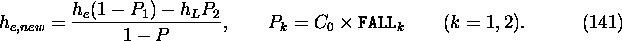If accepted, decide how many laser photons to absorb. To do so, sum up FINT(*,n,MY,j,l) from n=1 to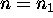until the sum becomes larger than. Then,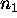will be the number of photons.

Onceis determined, the photon energy is determined by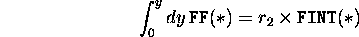where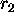is another uniform random number. The left hand side is known for the mesh point of y (i.e., FINT(k,n,MY,j,l)). Since we approximateby a piecewise linear function of y, the left hand side is a quadratic function between successive y's. Thus, inverse interpolation with respect to i by solving a quadratic equation gives the photon energy to be emitted.

The helicities of the final photon and electron are calculated from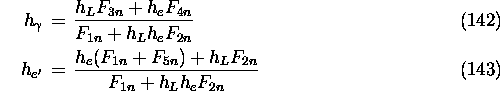for. This is done by directly calling a Bessel function routine.

Toshiaki Tauchi
Thu Dec 3 17:27:26 JST 1998